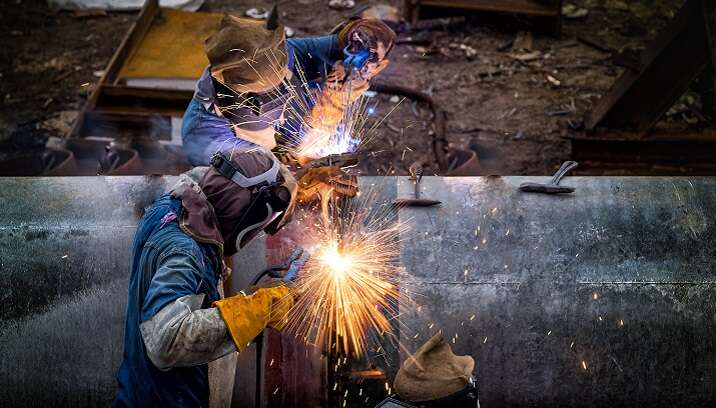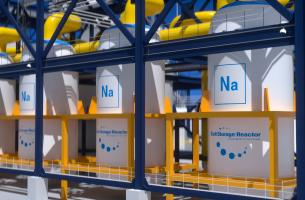# Carbon Equivalent fundamentals for predicting steel properties

When repairing a natural gas or oil pipeline, you need to ensure the new weld is absolutely solid. On a basic level, the integrity of the weld relies on the two pieces of pipe being completely compatible. If the two pieces of pipe behave differently, or become brittle during the welding process, the weld will fail – with potentially disastrous consequences.

So, you need to ensure you are using the right grade of steel. But relying on grade alone is risky, because even within a grade, the exact composition can vary enough to cause problems with weld integrity. This means you’ll have to measure the exact composition of each piece individually to fully understand how the component will behave.

This is where the carbon equivalent concept comes in. This allows you to take detailed material composition and turn that information into something useful that you can use to evaluate the weldability of the material.## Carbon equivalent is the key to predicting steel behavior

The equivalent carbon content concept is used on ferrous materials, typically steel and cast iron, to determine various properties of the alloy when more than just carbon is used as alloying elements.

It’s well understood that carbon levels within iron or steel affect their strength and brittleness and how the material can be worked and welded. However, carbon is not the only alloying element and other elements also contribute to material properties.

Considering the impact of each element and how it interacts with all the others individually would be difficult. So, instead the carbon equivalent concept is used where all the alloying elements are put through a formula that ‘converts’ them into an equivalent carbon percentage. The idea is to convert the percentage of alloying elements other than carbon to the equivalent carbon percentage, because the iron-carbon phases are better understood than other iron-alloy phases. This single number is then used to evaluate behavior, such as weldability as shown in the table below:

 Carbon equivalent (CE) Weldability Up to 0.35 Excellent 0.36–0.40 Very good 0.41–0.45 Good 0.46–0.50 Fair Over 0.50 Poor

You can see from the table that the larger the CE number, the poorer the weldability. So, how do we arrive at this number? That’s where the carbon equivalent equations come in:

1. International institute for welding (IIW) CE:
CE = (%C)+((%Mn)/6)+(((%Cr)+(%Mo)+(%V))/5)+(((%Cu)+(%Ni))/15)

2. Japanese welding institute PCM:
PCM = (%C)+((%Si)/30)+(((%Cr)+(%Cu)+(%Cr))/20)+((%Ni)/60)+((%Mo)/15)+((%V)/10)+((%B)*5)

3. Düren CEM:
CEM = (%C)+((%Si)/25)+(((%Mn)+(%Cu))/20)+(((%Cr)+(%V))/10)+((%Mo)/15) +((%Ni)/40)

4. Thyssen CET:
CET = (%C)+(((%Mn)+(%Mo))/10)+(((%Cr)+(%Cu))/20)+((%Ni)/40)

5. EN 10025-1 CEV:
CEV = (%C)+((%Mn)/6)+(((%Cu)+(%Ni))/15)+(((%Cr)+(%Mo)+(%V))/5)

We’ve presented five equations here; in practice you use the one that closely matches your type of steel.

You can see how each equation uses the percentage of carbon measured and then adds modified percentages of the other elements. For example, (%Mn / 6) which appears in the first equation, uses the percentage of manganese, but divides it by six which scales down its effect.

### Why are there several equations?

Over time, new equations were created to improve accuracy for different types of steel, such as low carbon. Also, they were extended to estimate the hydrogen cracking susceptibility of steel.

### Which one should you choose?

The first equation, adopted by the International Institute for Welding, is the most common. But if you are analyzing low carbon steel, the PCM and CEM expressions are more suitable. However, the second PCM equation is used for modern steels used in pipeline manufacture, where the carbon content is typically less than 0.11 wt.%.

### What do I actually need to measure in my pipe?

To accurately determine the weldability of the pipeline, you will need to measure the elements within the PCM equation:

Carbon, Silicon, Chromium, Copper, Nickel, Molybdenum, Vanadium and Boron.

You might also need to measure phosphorus and sulfur to give a more comprehensive analysis, and nitrogen will need to be measured if you need to identify duplex steels.

### Making CE calculations easy

Our range of spark OES instruments measure the elements necessary for accurate CE calculations and will go through the calculation and deliver the CE number.

Get in touch to discuss which one is right for you and to arrange a demo.

Get in touch

You might also be interested in:

### Author: Michael Molderings, Product Manager – Mobile OES